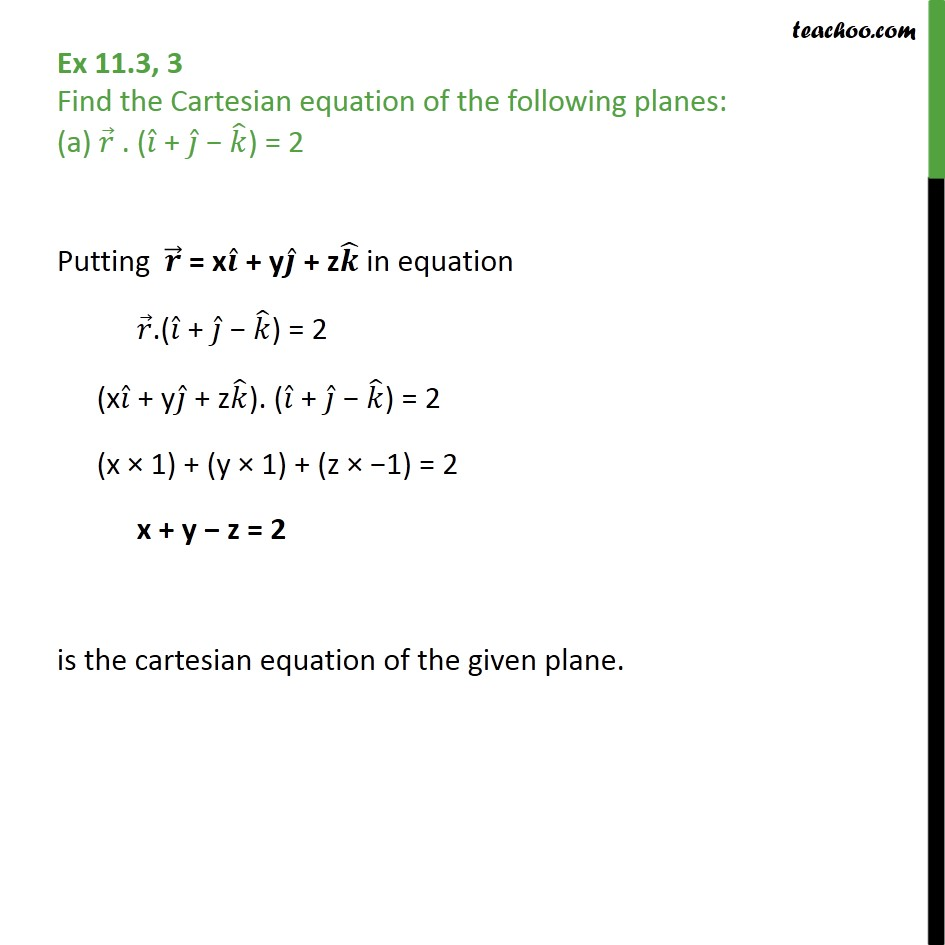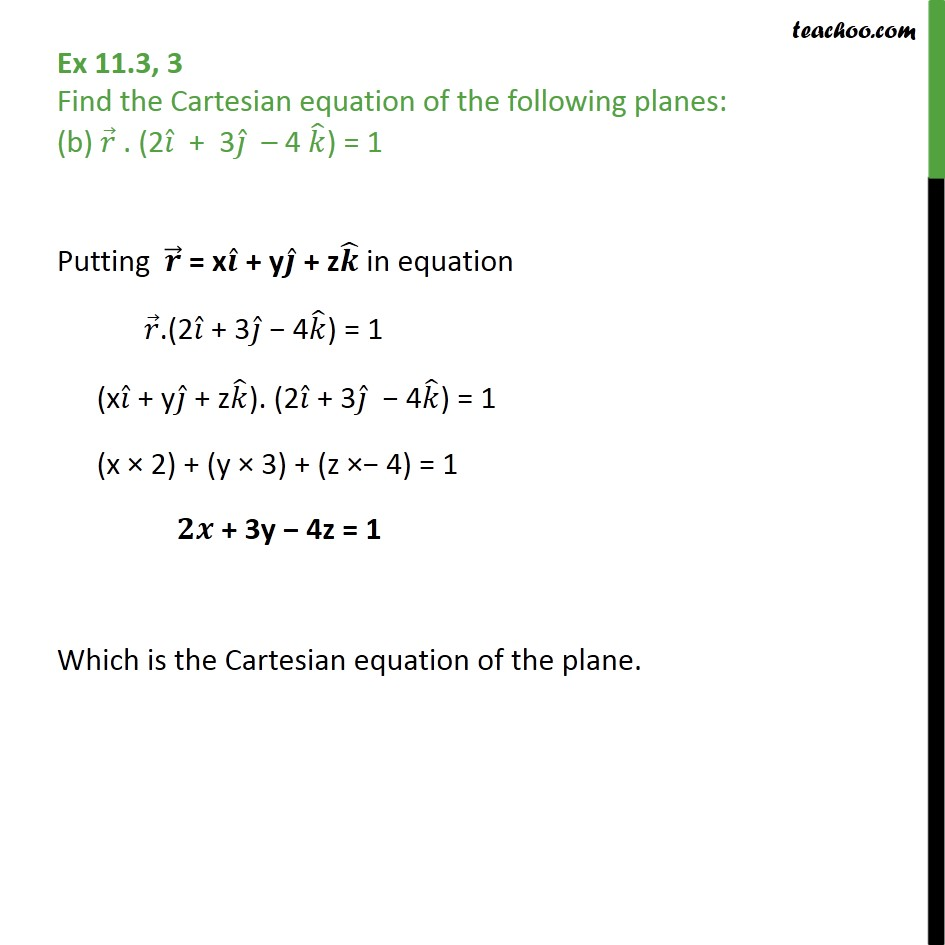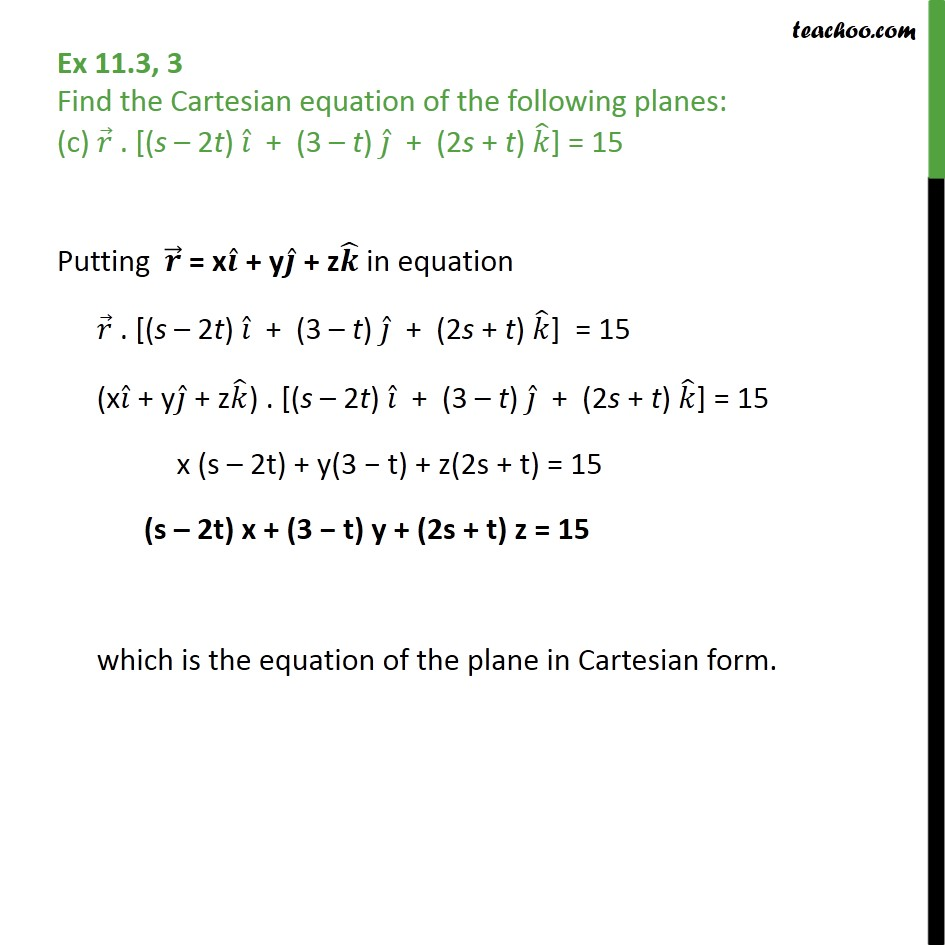Subscribe to our Youtube Channel - https://you.tube/teachoo

1. Chapter 11 Class 12 Three Dimensional Geometry
2. Serial order wise
3. Ex 11.3

Transcript

Ex 11.3, 3 Find the Cartesian equation of the following planes: (a) 𝑟﷯ . ( 𝑖﷯ + 𝑗﷯ − 𝑘﷯) = 2 Putting 𝒓﷯ = x 𝒊﷯ + y 𝒋﷯ + z 𝒌﷯ in equation 𝑟﷯.( 𝑖﷯ + 𝑗﷯ − 𝑘﷯) = 2 (x 𝑖﷯ + y 𝑗﷯ + z 𝑘﷯). ( 𝑖﷯ + 𝑗﷯ − 𝑘﷯) = 2 (x × 1) + (y × 1) + (z × −1) = 2 x + y − z = 2 is the cartesian equation of the given plane. Ex 11.3, 3 Find the Cartesian equation of the following planes: (b) 𝑟﷯ . (2 𝑖﷯ + 3 𝑗﷯ – 4 𝑘﷯) = 1 Putting 𝒓﷯ = x 𝒊﷯ + y 𝒋﷯ + z 𝒌﷯ in equation 𝑟﷯.(2 𝑖﷯ + 3 𝑗﷯ − 4 𝑘﷯) = 1 (x 𝑖﷯ + y 𝑗﷯ + z 𝑘﷯). (2 𝑖﷯ + 3 𝑗﷯ − 4 𝑘﷯) = 1 (x × 2) + (y × 3) + (z ×− 4) = 1 𝟐𝒙 + 3y − 4z = 1 Which is the Cartesian equation of the plane. Ex 11.3, 3 Find the Cartesian equation of the following planes: (c) 𝑟﷯ . [(s – 2t) 𝑖﷯ + (3 – t) 𝑗﷯ + (2s + t) 𝑘﷯] = 15 Putting 𝒓﷯ = x 𝒊﷯ + y 𝒋﷯ + z 𝒌﷯ in equation 𝑟﷯ . [(s – 2t) 𝑖﷯ + (3 – t) 𝑗﷯ + (2s + t) 𝑘﷯] = 15 (x 𝑖﷯ + y 𝑗﷯ + z 𝑘﷯) . [(s – 2t) 𝑖﷯ + (3 – t) 𝑗﷯ + (2s + t) 𝑘﷯] = 15 x (s – 2t) + y(3 − t) + z(2s + t) = 15 (s – 2t) x + (3 − t) y + (2s + t) z = 15 which is the equation of the plane in Cartesian form.

Ex 11.3